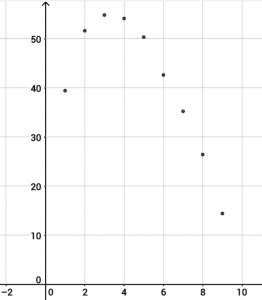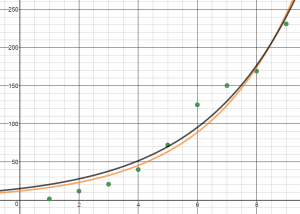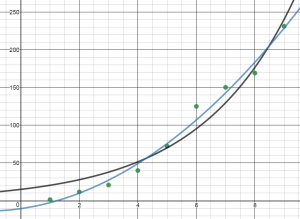# Equations from Data

## Equations from Data

When businesses, governments and other policy makers prepare to make decisions, they often look at data to find trends. In this activity, we will use data and a graphing calculator, Desmos, or Geogebra to find an equation that best fits the data points.

Statista has Global Apple iPod sales (in million units). Plot the data in a table in Desmos, a spreadsheet in Geogebra, or on your graphing calculator (click the links for instructions). When you enter your data, let the first year have the value x = 1 not x = 2007.

When you find a regression model for your data, an R2 value that is close to 1 shows a strong correlation between your data and your regression model (line, or curve). You can make projections about iPod sales using the regression equation.

1. Why did iPod sales peak in 2009 and then start falling?
2. What is your regression equation?
3. Based on your model, when do you predict iPods will no longer be sold?
4. Is your answer to question 3 likely or is it possible that there will always be a market for iPods? Explain.Statista also lists Unit sales of the Apple iPhone worldwide (in millions). Plot the data in a table in Desmos, a spreadsheet in Geogebra, or on your graphing calculator (click the links for instructions). This data appears to more closely resemble an exponential growth model of the form y=abx.

2. Based on your model, how many iPhones do you predict will be sold in 2018?4. Is there a better model for this data than exponential growth?
5. If so, what is it and what is the regression equation?6. How does that affect your predictions for sales for the next two years?

## Chapter 3 Project